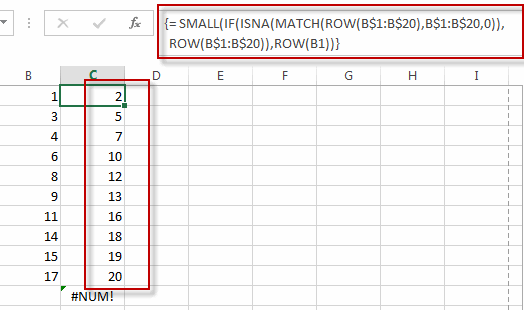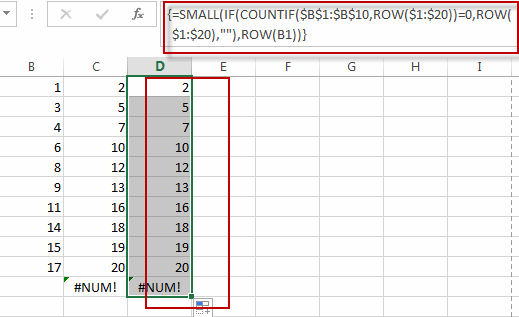# Find Missing Numbers in a Sequence in Excel

This post will guide you how to find missing numbers in a sequence with a formula in Excel. How do I identify missing numbers in a consecutive series in Excel. How to find missing serial number in Excel 2013/2016.

## Find Missing Numbers in a Sequence in Excel

Assuming that you have a serial number list in Column B, and you want to find the missing number in this sequence list. How to achieve it.

You can use an excel array formula based on the SMALL function, the IF function, the ISNA function, the MATCH function, and the ROW function. Like this:

=SMALL(IF(ISNA(MATCH(ROW(B\$1:B\$20),B\$1:B\$20,0)),ROW(B\$1:B\$20)),ROW(B1))

Type this array formula into a blank cell, and then press Ctrl + Shift + Enter keys in your keyboard.The missing numbers are listed in cells.

Note: this formula will check the given sequence from 1 to 20 if there are missing numbers. If so, returns the missing numbers.

Or you can use another array formula based on the Small function, the IF function, the Countif function and the row function to achieve the same result. Like this:

=SMALL(IF(COUNTIF(\$B\$1:\$B\$10,ROW(\$1:\$20))=0,ROW(\$1:\$20),””),ROW(B1))### Related Functions

• Excel MATCH function
The Excel MATCH function search a value in an array and returns the position of that item.The syntax of the MATCH function is as below:= MATCH  (lookup_value, lookup_array, [match_type])….
• Excel SMALL function
The Excel SMALL function returns the smallest numeric value from the numbers that you provided. Or returns the smallest value in the array.The syntax of the SMALL function is as below:=SMALL(array,nth) …
• Excel ROW function
The Excel ROW function returns the row number of a cell reference.The ROW function is a build-in function in Microsoft Excel and it is categorized as a Lookup and Reference Function.The syntax of the ROW function is as below:= ROW ([reference])….
• Excel IF function
The Excel IF function perform a logical test to return one value if the condition is TRUE and return another value if the condition is FALSE. The IF function is a build-in function in Microsoft Excel and it is categorized as a Logical Function.The syntax of the IF function is as below:= IF (condition, [true_value], [false_value])….
• Excel COUNTIF function
The Excel COUNTIF function will count the number of cells in a range that meet a given criteria. This function can be used to count the different kinds of cells with number, date, text values, blank, non-blanks, or containing specific characters.etc.= COUNTIF (range, criteria)…

Related Posts

How to Sum the Smallest N Values in Excel

Sometimes we may want to sum the first smallest N numbers in a range in Excel. In this article, we will show you the method of “SUM the Smallest N Numbers” by a simple formula which consist of SUMPRODUCT and ...

How to Create Dynamical Drop-Down List and Sort by Alphabetical Order in Excel

In our daily work we may need to create a dynamical dropdown list and sort all values by alphabetical order. To create a dropdown list like this, we need to apply some built-in features like ‘Define Name’ and ‘Data Validation’ ...

How to Find the Smallest Value and Smallest Positive Value in Excel

Sometimes we want to find out the smallest positive value among a set of values. We should ignore the negative value and zero value when getting the smallest positive value. So we design different cases in this article to demonstrate ...

How to Convert Multiple Rows into a Single Row in Excel

This post will guide you how to convert multiple rows or columns into a single row with a formula in Excel 3013/2016. How do I convert groups of rows to a single row of columns with VBA Macro in Excel. ...

How to Remove Numbers from Text in Excel

This post will guide you how to remove all numbers from a text string in one cell in Excel. How do I strip all numeric characters from a given cell with a formula in Excel. How do I remove numbers ...

How to Highlight Every Other Row Using Conditional Formatting in Excel

This post will guide you how to highlight every other row in Excel. How do I shade every other row with conditional formatting in Excel. Highlight Every Other Row Video: Highlight Every Other Row Highlight Every Other Row Assuming that ...

How to Get the First, 2nd or Nth Match Using Vlookup/Index/Match

This post will guide you how get the nth matching values with VLOOKUP function in Excel. How do I find the nth match value with Index/Match formula in Excel. How to find the first, second, third or nth matching value ...

Ignoring Blank or Zero Cells with Conditional formatting

This post will guide you how to make conditional formatting ignore blank cells or zero cell in Excel. How do I force blank cells or zero cells to be ignored in conditional formatting in Excel. How to ignore blank cells ...

Find the Earliest and Latest Date in a Range of Dates in Excel

This post will guide you how to find the earliest date in a range of dates in Excel. How do I get the earliest and latest date in a range with a formula in Excel. How to find the highest ...

Find Closest Value or Nearest Value in a Range in Excel

This post will guide you how to find the closest value or nearest value in a range of cells or in a column in Excel. How do I find the closest match in a range to a given lookup value ...

Sidebar